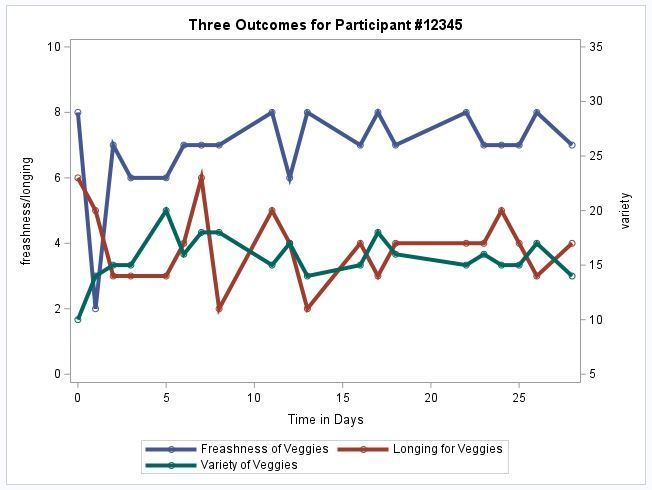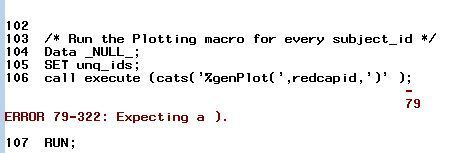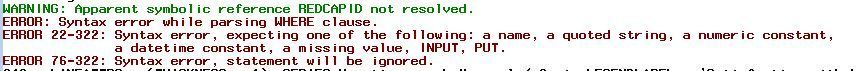## PROC SGPLOT one command to produce graph for each case? And, question about "inset" possibilities

Greetings!

Two questions related to PROC SGPLOT.

I would like to produce one graph for each case in my data set.

Here is the code I'm using. I'm running this code once for each case. My first question is, is there one command so that sas will produce one graph for each case without my having to copy and paste 100 versions of this code? Here is the code:

``````PROC SGPLOT DATA = filename;
where subject_id=12345;
SERIES X = time_graph Y = v1 / LEGENDLABEL = 'Freashness of Veggies'
MARKERS LINEATTRS = (THICKNESS = 4);
SERIES X = time_graph Y = v2 / LEGENDLABEL = 'Longing for Veggies'
MARKERS LINEATTRS = (THICKNESS = 4);
SERIES X = time_graph Y = v3 /y2axis LEGENDLABEL = 'Variety of Veggies'
MARKERS LINEATTRS = (THICKNESS = 4);
yaxis min=0 label='freashness/longing' values=(0 to 10 by 2);
y2axis min=5 label='variety' values=(5 to 35 by 5);
TITLE 'Three Outcomes for Participant #12345';
RUN;``````

Here is the nice graph it produces:My second question is: I'd love to use the "inset" feature to print on each graph specific info for each case, for example, that individual's weight and height.

Can the "inset" feature do this and if so, what would the code look like?

Thank you for your patience because I am a new user still finding my way.

Much appreciated and eager to hear what you think!

1 ACCEPTED SOLUTION

Accepted Solutions

## Re: PROC SGPLOT one command to produce graph for each case? And, question about "inset" po

Hi,

As was mentioned, BY-group processing in SAS is a very powerful feature.  I don't think you can get the INSET statement to work with by-group processing, but there are other options.  If you are ok with the height and weight being listed in the title, then you can get one plot per participant with a simple step:

``````TITLE 'Three Outcomes for Participant #ByVal1 (height: #ByVal2 weight: #ByVal3)' ;

PROC SGPLOT DATA = filename;
by subject_id height weight;
SERIES X = time_graph Y = v1 / LEGENDLABEL = 'Freashness of Veggies'
MARKERS LINEATTRS = (THICKNESS = 4);
SERIES X = time_graph Y = v2 / LEGENDLABEL = 'Longing for Veggies'
MARKERS LINEATTRS = (THICKNESS = 4);
SERIES X = time_graph Y = v3 /y2axis LEGENDLABEL = 'Variety of Veggies'
MARKERS LINEATTRS = (THICKNESS = 4);
yaxis min=0 label='freashness/longing' values=(0 to 10 by 2);
y2axis min=5 label='variety' values=(5 to 35 by 5);
RUN;

title ;``````

This assumes that height and weight are constant for a participant.

If you really want the value to be inside the graph, there are some other approaches that would work.

This blog post has more examples of this approach:

https://blogs.sas.com/content/iml/2021/02/22/byvar-byval-keywords-sas-titles.html

Check out the Boston Area SAS Users Group (BASUG) video archives: https://www.basug.org/videos.
14 REPLIES 14AhmedAl_Attar
Rhodochrosite | Level 12

## Re: PROC SGPLOT one command to produce graph for each case? And, question about "inset" po

I don't have an answer for your inset question, but I can show how to automate the Graph generation 😊

``````%macro genPlot(subjectid);
PROC SGPLOT DATA = filename;
where subject_id=&subjectid;
SERIES X = time_graph Y = v1 / LEGENDLABEL = 'Freashness of Veggies'
MARKERS LINEATTRS = (THICKNESS = 4);
SERIES X = time_graph Y = v2 / LEGENDLABEL = 'Longing for Veggies'
MARKERS LINEATTRS = (THICKNESS = 4);
SERIES X = time_graph Y = v3 /y2axis LEGENDLABEL = 'Variety of Veggies'
MARKERS LINEATTRS = (THICKNESS = 4);
yaxis min=0 label='freashness/longing' values=(0 to 10 by 2);
y2axis min=5 label='variety' values=(5 to 35 by 5);
TITLE 'Three Outcomes for Participant #12345';
RUN;
%mend genPlot;
/* Get table of unique subject_ids */
PROC SORT DATA=filename(KEEP=subject_id) OUT=unq_ids NODUPKEY;
BY subject_id;
RUN;

/* Run the Plotting macro for every subject_id */
Data _NULL_;
SET unq_ids;
call execute (cats('%genPlot(',subjectid,')' );
RUN;
``````

IF you get an answer to your "inset" question, you may need to update Proc SGplot once only.

Hope this helps

## Re: PROC SGPLOT one command to produce graph for each case? And, question about "inset" po

Thank you!

I did get an error message--I tried to repair it by adding a ')' where indicated, but that didn't work. Please advise!AhmedAl_Attar
Rhodochrosite | Level 12

## Re: PROC SGPLOT one command to produce graph for each case? And, question about "inset" po

try changing it to
call execute ('%genPlot('|| STRIP(PUT(subjectid,best.)) || ')' );

## Re: PROC SGPLOT one command to produce graph for each case? And, question about "inset" po

That also resulted in an error term.It seems to still be unhappy with the call execute line.

(redcapID is the actual studyid, I changed it in every case.)

Would be glad to try something else if you'd be willing!AhmedAl_Attar
Rhodochrosite | Level 12

## Re: PROC SGPLOT one command to produce graph for each case? And, question about "inset" po

Can you show us your final modified code?AhmedAl_Attar
Rhodochrosite | Level 12

## Re: PROC SGPLOT one command to produce graph for each case? And, question about "inset" po

Try

``call execute (cats('%genPlot(', redcapid, ')'));``

## Re: PROC SGPLOT one command to produce graph for each case? And, question about "inset" po

Well, you could look at doing

``by case;``

With case being a variable which splits the graph.

But your second question may not work then.  Behind the scenes sgplot and others create graph template language, you can see this by updating your code:

``PROC SGPLOT DATA = filename tmplout="c:\gtl_code.txt";``

The file c:\gtl_code.txt will show you what GTL is created by your sgplot.  I mention this as it gives a fair bit more freedom, for instance you can have dynamic variables and all kinds of conditionals.

In terms of your specific part, I would highly recommend reading through the thousands of examples in:

https://blogs.sas.com/content/graphicallyspeaking/

Its the best resource for all graphing, with examples in sgplot, gtl.  You will likely find something similar to what you want and can start with that.

## Re: PROC SGPLOT one command to produce graph for each case? And, question about "inset" po

Hi,

As was mentioned, BY-group processing in SAS is a very powerful feature.  I don't think you can get the INSET statement to work with by-group processing, but there are other options.  If you are ok with the height and weight being listed in the title, then you can get one plot per participant with a simple step:

``````TITLE 'Three Outcomes for Participant #ByVal1 (height: #ByVal2 weight: #ByVal3)' ;

PROC SGPLOT DATA = filename;
by subject_id height weight;
SERIES X = time_graph Y = v1 / LEGENDLABEL = 'Freashness of Veggies'
MARKERS LINEATTRS = (THICKNESS = 4);
SERIES X = time_graph Y = v2 / LEGENDLABEL = 'Longing for Veggies'
MARKERS LINEATTRS = (THICKNESS = 4);
SERIES X = time_graph Y = v3 /y2axis LEGENDLABEL = 'Variety of Veggies'
MARKERS LINEATTRS = (THICKNESS = 4);
yaxis min=0 label='freashness/longing' values=(0 to 10 by 2);
y2axis min=5 label='variety' values=(5 to 35 by 5);
RUN;

title ;``````

This assumes that height and weight are constant for a participant.

If you really want the value to be inside the graph, there are some other approaches that would work.

This blog post has more examples of this approach:

https://blogs.sas.com/content/iml/2021/02/22/byvar-byval-keywords-sas-titles.html

Check out the Boston Area SAS Users Group (BASUG) video archives: https://www.basug.org/videos.

## Re: PROC SGPLOT one command to produce graph for each case? And, question about "inset" po

Very helpful! I got an error message saying "data set [name of data set] is not sorted in ascending sequence."

## Re: PROC SGPLOT one command to produce graph for each case? And, question about "inset" po

@BalletYoga wrote:

Very helpful! I got an error message saying "data set [name of data set] is not sorted in ascending sequence."

When you're using a BY statement, the data must be sorted in the order of the BY variables:

``````proc sort data=mydata;
by subjectid;
run;``````

But if you're using that approach, please see @Rick_SAS 's answer before mine.  He added some stuff I forgot, like options nobyline.  If I had seen his answer, I wouldn't have written mine.  I was just too slow in writing my answer (and stole from his blog anyway. : )

Check out the Boston Area SAS Users Group (BASUG) video archives: https://www.basug.org/videos.

## Re: PROC SGPLOT one command to produce graph for each case? And, question about "inset" po

It was very hard to choose one helper as the solution, because I drew from recommendations from everyone.

The whole experience was magical.

You will see I added some flourishes, but this is the final code that worked beautifully:

``````PROC SGPLOT DATA = dataset;
by subject_id tomato notsorted cucumber notsorted;
ods graphics / width=300px height=250px;
SERIES X = time_graph Y = fresh / LEGENDLABEL = 'Fresh'
MARKERS LINEATTRS = (THICKNESS = 4);
SERIES X = time_graph Y = desire / LEGENDLABEL = 'Desire'
MARKERS LINEATTRS = (THICKNESS = 4);
SERIES X = time_graph Y = local /y2axis LEGENDLABEL = 'local'
MARKERS LINEATTRS = (THICKNESS = 4);
yaxis min=0 label='fresh/desire' values=(0 to 10 by 2);
y2axis min=5 label='local' values=(5 to 35 by 5);
xaxis display=(nolabel) offsetmin=0.05 offsetmax=0.05 values=(0 to 28 by 1);
format time_graph myxaxis. ;
options nobyline;
TITLE "Outcomes for Participant #ByVal1 #ByVal2 #ByVal3";
RUN;``````

## Re: PROC SGPLOT one command to produce graph for each case? And, question about "inset" po

1. Sort the data by the subject_id variable and replace the WHERE statement with a BY statement:
BY subject_id;

2. I don't think there is support for an inset to use values of a BY variable. The traditional way to do this is in a title statement. Read about "How to use the #BYVAR and #BYVAL keywords to customize graph titles in SAS."

Here's an example with some fake weight and height values.

``````data Have;
set Sashelp.Stocks;
where '01Jan1998'd <= Date <= '30May2000'd;
/* prepare data to display information */
if      Stock='IBM'       then do; Weight=180; Height = "5'6"; end;
else if Stock='Intel'     then do; Weight=190; Height = "5'6"; end;
else if Stock='Microsoft' then do; Weight=160; Height = "5'7"; end;
run;
/* NOTE: The example data set is already sorted by Stock and by Date.
Be sure to sort your data if you want to use the BY statement. For example:
proc sort data=Have;
by Stock Date;
run;
*/
options nobyline;                                /* suppress automatic title */
title "Data for #byval1";
title2 "Weight=#byval2; Height=#byval3";
proc sgplot data=Have;
by Stock Weight notsorted Height notsorted;
series x=Date y=Open / lineattrs=(thickness=2);
yaxis grid label="Stock Price"; /* optional: min=50 max=210 */
xaxis display=(nolabel);
run;
``````

## Re: PROC SGPLOT one command to produce graph for each case? And, question about "inset" po

In SAS almost any time you want something done per level of a variable, or combinations of levels, the first thing to think of is BY group processing.

If you data is sorted by subject_id then:

```options NOBYLINE;
PROC SGPLOT DATA = filename;
BY subject_id=12345;
SERIES X = time_graph Y = v1 / LEGENDLABEL = 'Freashness of Veggies'
MARKERS LINEATTRS = (THICKNESS = 4);
SERIES X = time_graph Y = v2 / LEGENDLABEL = 'Longing for Veggies'
MARKERS LINEATTRS = (THICKNESS = 4);
SERIES X = time_graph Y = v3 /y2axis LEGENDLABEL = 'Variety of Veggies'
MARKERS LINEATTRS = (THICKNESS = 4);
yaxis min=0 label='freashness/longing' values=(0 to 10 by 2);
y2axis min=5 label='variety' values=(5 to 35 by 5);
TITLE "Three Outcomes for Participant # #bval";
RUN;Options byline;```

BY group processing typically has a line like Subject_id=12345 above each item created. The OPTIONS NOBYLINE suppresses that. There are automatic variables associated with the BY line, #byval references the current value of the By variable. I placed a space in the code to separate your # for "number" from the # part of Byval syntax that tells SAS to use that special value. There is also a #byvar for the variable name. And since you can have multiple variables on a BY statement there is syntax to reference each variable and value separately by suffixing with the position of the variable on the by statement.

If your data is not sorted by the By variables but is grouped together you add the NOTSORTED option to the By statement.

Where is your data for the "individual's height and weight"?

If you have a the values in variables, one per subject_id then probably a TEXT plot might be easiest, or possibly an XAXISTABLE.

From SAS Users blog